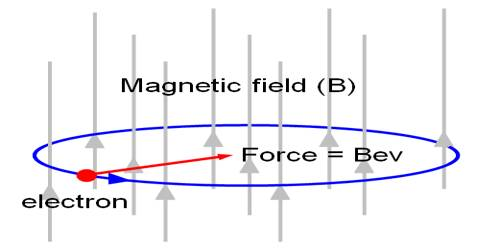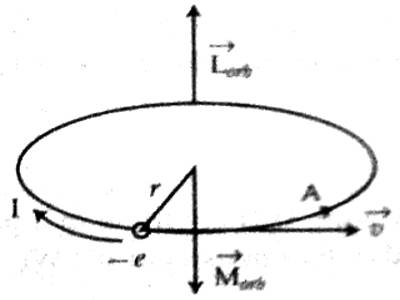# Moving Electron in an Orbit

Moving Electron in an orbit

We know, electrons revolve in fixed orbits outside the centre of an atom. Due to this orbital motion of the electrons, a magnetic moment is associated with each electron. Diamagnetism originates in materials due to this orbital motion in the atom, so diamagnetism is present in all materials. But its influence is very weak. Materials which are formed by atoms of net magnetic moment i.e. in those materials which show paramagnetic and ferromagnetism, although diamagnetism exists in these materials due to weakness its influence is not evident.Suppose, an electron is revolving anti-clockwise in the orbit of the atom with velocity v and radius r [Figure]. This motion of electrons can be compared to conventional current (I). Due to the motion in circular orbit electrons have orbital angular momentum Lσrb and a magnetic dipole moment Mab. In this case duration of rotation of electron is opposite to the flow of current. Orbital magnetic dipole moment for an electron rotating in an orbit or for a current loop is,

Mσrb = IA … … …. (1)

Here A = area of the closed loop.

The direction of this magnetic dipole moment can be determined by right hand rule. If time taken for an electron of negative charge e for complete circular path is, T = 2πr/v, then current, I = charge/time = e / (2πr/v) … … … (2)

From the equation (1) we get,

Mσrb = e / (2πr/v) x πr2

Mσrb = evr / 2 … … … (3)

Since r and v are perpendicular to each other, orbital angular momentum.

Lσrb = m (r x v)

or, Lσrb = mrv sin 900 = mrv … …. (4)

From equations (3) and (4) it can be written as,

Mσrb = – (e/2m) Lσrb;

The negative sign means M and L are opposite to each other. The same result is found in case of quantum mechanics.